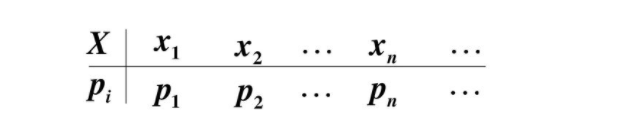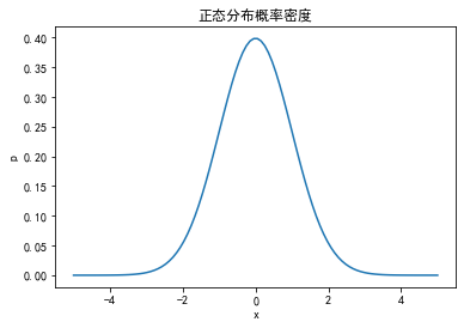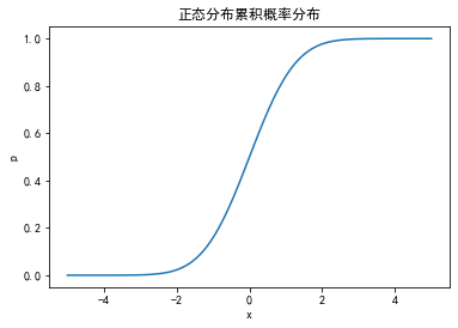2 篇文章 0 订阅

# 理解3：# PS：python中使用

## 概率密度函数使用

python中可以使用科学计算包scipy中的统计模块实现概率密度函数，分布函数的计算，计算前可以对数据进行标准化处理，然后标准正态分布在某个点的概率密度可用scipy.stats.norm.pdf计算。借用别人的代码绘图正态分布：

from scipy import stats
import numpy as np
import matplotlib.pyplot as plt
plt.rcParams['font.sans-serif']=['SimHei']#用来正常显示中文标签
plt.rcParams['axes.unicode_minus']=False#用来正常显示负号
#正态分布概率密度
X = []
Y = []
for a in np.linspace(-5, 5, 100):
y = stats.norm.pdf(a)
X.append(a)
Y.append(y)
plt.plot(X, Y)
plt.xlabel("x")
plt.ylabel("p")
plt.title("正态分布概率密度")
plt.show()## 分布函数使用

from scipy import stats
import numpy as np
import matplotlib.pyplot as plt
plt.rcParams['font.sans-serif']=['SimHei']#用来正常显示中文标签
plt.rcParams['axes.unicode_minus']=False#用来正常显示负号
#正态分布概率密度
X = []
Y = []
for a in np.linspace(-5, 5, 100):
y = stats.norm.cdf(a)
X.append(a)
Y.append(y)
plt.plot(X, Y)
plt.xlabel("x")
plt.ylabel("p")
plt.title("正态分布累积概率分布")
plt.show()## 产生正态分布样本

import numpy as np
#设置随机数种子seed
np.random.seed(456789)
#生成15个均值为10，标准差为2的正态分布样本
r = np.random.normal(loc=10, scale=2, size=15)
print(r)

09-1110万+06-23982
10-314万+
08-311万+
03-137952
11-018053
06-11382
12-191万+
03-12392
05-064088
07-231380
05-212万+
05-08507
07-069258
04-21548
08-259万+
06-10875
06-148031
03-1912万+点击重新获取扫码支付余额充值